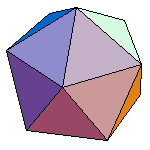index: click on a letter A B C D E F G H I J K L M N O P Q R S T U V W X Y Z A to Z index index: subject areas numbers & symbols sets, logic, proofs geometry algebra trigonometry advanced algebra & pre-calculus calculus advanced topics probability & statistics real world applications multimedia entrieswww.mathwords.com about mathwords website feedback

Platonic Solids
Regular Polyhedra

The solids which have faces that are all congruent regular polygons and which has dihedral angles that are all congruent.

There are only five possible shapes for a regular polyhedron: regular tetrahedron, cube, octahedron, dodecahedron, and icosahedron.

 Tetrahedron Cube Octahedron Dodecahedron IcosahedronIf your browser is Java-enabled, try dragging, shift dragging, control dragging, and right-click dragging both vertically and horizontally across these figures.